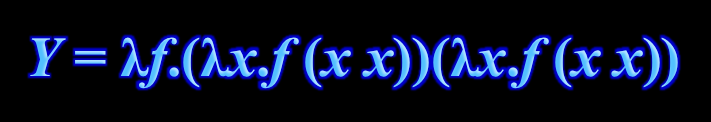# Round 8: Recursion(Curry’s Y combinator.)

Learning objectives

In this round you will learn …

• … that recursion is one of the basic programming principles to organize computations and data

• … that the power of recursion stems from controlled self-reference that terminates at base cases

• in essence, we can implement a large computation (or data structure)

using smaller, self-similar, parts until the computation is so small so as to become trivial

• … that many familiar mathematical objects and functions benefit from a recursive definition

• for example, a string of length $$n$$

either (i) is empty (when $$n=0$$, the base case), or (ii) consists of a first character followed by a string of length $$n-1$$ (when $$n\geq 1$$, the recursive case)

• recursive definitions lead to simple recursive functions that manipulate data or carry out a computation

• recursive definitions admit mathematical analysis via mathematical induction (*)

• … that recursion is naturally associated with a recursion tree that records all the stages of recursion

• … that recursion is a natural tool to carry out exhaustive search

• … how to use tail recursion to obtain efficiency

(Material that is marked with one or more asterisks (*) is good-to-know, but not critical to solving the exercises or passing the course.)